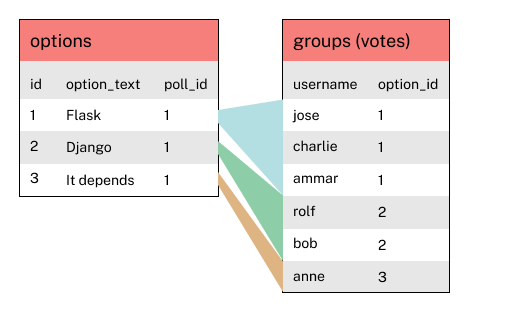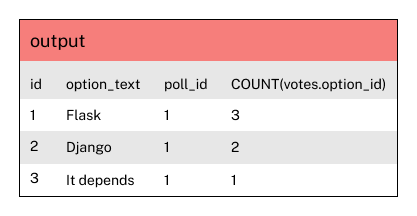# # PostgreSQL window functions (part 2)

For this chapter, let's go back to our polling application data.

Below is the data set we're using in this chapter. You can also see this in an interactive database fiddle.

polls

2 Python vs. Java rolf
3 Windows vs. Mac bob

options

id option_text poll_id
2 Django 1
3 It Depends 1
4 Python 2
5 Java 2
6 Windows 3
7 Mac 3

jose 1
charlie 1
ammar 1
rolf 2
bob 2
anne 4
eric 4
jose 4
charlie 4
ammar 4
rolf 4
bob 4
anne 5
eric 5
bob 4
anne 6
eric 7

## # Why we need a window function in our vote percentages query

In our query earlier, we had something like this:

``````SELECT
options.id,
options.option_text,
FROM options
WHERE options.poll_id = 1
GROUP BY options.id;
``````

Aggregate functions like `COUNT` and `SUM` get calculated while the `GROUP BY` is being evaluated. For example, in `COUNT(votes.option_id) as vote_count` the value increases as the query processes each group, until it finishes.

That's why you can't have nested aggregate functions: you can't `SUM` something that is still being calculated.

However, window functions run after the `GROUP BY` and aggregate functions are evaluated. When the `SUM() OVER()` part of `SUM(COUNT(votes.option_id)) OVER()` runs, the `COUNT()` has already been calculated.

Let's take this step by step, and look at what the result set evaluates to before running the window function.

We've got a `FROM options`, a `LEFT JOIN` with `votes`, a `WHERE` clause, and a `GROUP BY`. For the purposes of these diagrams, I'm going to ignore any `SELECT` columns since those are processed at the very end.

Let's first of all create a diagram of what the rows and groups look like:Then, aggregate functions run before window functions. With `SUM(COUNT(votes.option_id)) OVER()`, it means that `COUNT(votes.option_id)` runs first, so the window function 'sees' and can operate on this:And then `SUM()` runs on that, giving us the total number of votes.

So to recap, the important part here is that `SUM(COUNT())` look nested in the code, but they aren't really. The are evaluated at different points, so both functions are just operating on a column of data.

We can further break down the data that the window function operates on by placing stuff inside the brackets of `OVER()`. Let's take a look at an example.